## Integral of Action with the Ghosts

Chubykalo A

Espinoza A

Korneva M

Physical faculty Department of an electronics, Voronezh State University, Russia

Article
Article Info
Author Info
Figures & Data

## Abstract

The variants of the implementation of the principle of least action in relativistic mechanics are analyzed. It is shown that the relativistic integral of the action has no extremes. The value of the integral is constant and depends only on the limits of integration. It is shown that the principle of least action cannot be implemented in the Minkowsky space due to the dependence of ds on dxi. An inaccuracy made by A. Einstein in explaining mental experiments is described. “Compression scales” and “time dilation”, apparently, do not exist. To search for equations of motion, it is necessary to back to the classical principle of least action.

## Keywords

Action Integral; The Equation of Motion

## Introduction

More than 100 years have passed since the crisis of physics. The main idea of the crisis was the thesis that classical mechanics was “outdated” and it was necessary to create a new “relativistic” mechanics . At that time it was necessary to solve many problems urgently, and physicists were in a hurry. Some questions they had not thought out deeply. Physicists hoped that the new generation would refine the results obtained by them.

As an example, we cite the expression of N. Bohr, which we encountered in . According to N. Bohr, “the book of Landau and Lifshitz ‘Field Theory’  was written in the spirit of early logical positivism.” Physicists do not really respect philosophy. Therefore, we decipher this statement. Logical positivism, on the one hand, demanded external rigor of presentation of the material, on the other hand, demanded to hide internal contradictions in scientific theories and “justify” incorrect results. You will find this out if you compare the style of presentation in  with the “Feynman Lectures in physics” .

This article discusses errors made in the implementation of the mathematical formalism of the relativistic variational principle, i.e. principle of least action for relativistic mechanics.

#### Classic Action Integral

The relativistic principle of least action was built in the image and likeness of the classical principle of least action for mechanics. Therefore, let us recall briefly the basis of the classical principle of least action.

1. A classical action integral is introduced.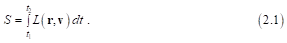1. Integration is carried out onfrom t1 to t2
2. The Lagrange function L(r, v) is equal to the difference between the kinetic and potential energy of the particle.
3. It depends on the coordinates and velocities of the particle, but does not explicitly depend on time. Therefore, the action integral is a functional.
4. We are looking for such a trajectory so that when integrating along it the integral of the action S takes the minimum value. To this end, we vary the coordinates and velocities of the particle. The time does not vary (δt = 0).
5. Variation of coordinates and velocities (δr, δv) in the integrand L(r, v) allows finding the differential equation of the trajectory, integration along which realizes the minimum of the integral of the action.
6. The variation of the integral is zero δS = 0 when integrated along an extremal, i.e. straight line satisfying the “equation of motion of a particle.”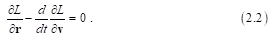There is the Noether theorem that allows us to find the conservation laws (first integrals) for a particle moving in a force field: the law of conservation of momentum, the law of conservation of energy, the law of conservation of angular momentum.

#### The First Option of the Relativistic Integral

Integral of action. (The first variant is stated in ) “Having recognized” the classical mechanics as obsolete, scientists had to build something new. They began to build the mathematic formalism of relativistic mechanics in a manner similar to the classical one. Scientists have not seen the other way. It was necessary to formulate in a new way the principle of least action in the framework of relativistic ideas. The criterion for the correctness of the results served as the principle of conformity. When v≪c with the mathematical formalism of the principle of least action of relativistic mechanics had to go into the mathematical formalism of the principle of least action of classical mechanics.

The relativistic integral of the action in the first variant resembles the classical integral of action (2.1).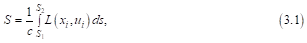where L is the Lagrange function for a particle on which an external field acts, c is the speed of light, xi is the 4-coordinate of the particle (ict,x,y,z), ui is the 4-velocity of the particle: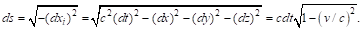It is known that the 4-coordinate xi depends on s, and when differentiating it by and we can look at its content with a specific example s, we have the 4-velocity of the particle: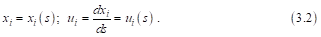So, the parameter s is similar to the parameter t in classical mechanics . When varying the integral of the action, it, like t, remains unchanged (δds=0). The condition δds=0 is crucial for the first option. As a result, we have the equation of motion of a particle in the form that fully corresponds to the classical equation (2.2)So, the external form is realized,  and we can look at its content with a specific example.

An example. The author  proposes the following expression of the Lagrange function for a charge in an electromagnetic field: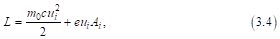where e and mo are the charge and charge mass, respectively, ui is the 4-velocity of the particle, Ai is 4-potential of the electromagnetic field. The first term in expression (3.4) is kinetic energy. Its classic counterpart is (m0v2)/2. The second term in expression (3.4) is the charge interaction energy with the electromagnetic 4-potential.

By varying the integral of the action by standard methods, it is easy to find the following equation of motion for charge:This is the relativistic equation of motion (the Lorentz formula), which, with V = C, transforms into the well-known classical equation:where A and φ  are the potentials of the electromagnetic field, V is the charge velocity.

It would seem that we correctly described the relativistic principle of least action, but careful analysis reveals several problems.

Problem 1.  At low speeds (V = C) we do not get the classical Lagrange function. In SRT there is an identity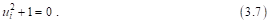Taking this relationship into account, it can be shown that expression (3.7) actually does not correspond to its analogue (3.4). It has the form in the "classic version":The kinetic energy of the particle has disappeared!

Problem 2. Moreover, using expression (3.7) we can write many other new Lagrange functions, which are equal to the Lagrange function (3.4). From them we can get many other different equations of motion.  For example, using the identity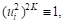we can write the expression for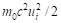in formula (3.4) in the following formwhere K is a positive integer (K =0,1,2). It’s easy to make sure that instead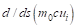we obtain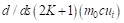.

In the expression for the Lagrange function (3.4) we can add the “zero term”, for example,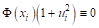. Then in the equation of motion there will appear an “additive”, different from zero: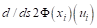etc. All these “additives” we will call "ghosts." Now we can no longer be sure that the Lorentz formula (3.5) is unique and does not contain hidden “ghosts” inside!

So, we can get many different equations of motion by changing the values of K and Φ. In this case, the Lagrange function will actually be the same! Why does this variety of equations involving ambiguity take place, and which particular equation of motion from this set is correct (true)?

Perhaps this is due to the fact that the variable s in SRT cannot be considered as an independent variable like t in Newtonian mechanics. Indeed, on the one hand, s depends on xi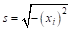on the other hand, must depend xi on. Due to this, the conditions for the application of the calculus of variations are violated (we recall that δds = 0). For this reason, the variant considered above cannot serve as the basis for the mathematical formalism of the STR, which implements the principle of least action.

#### The Second Variant of the Relativistic Integral

The second variant. Another version of the action integral is given in the textbook . The authors of  are well aware that s depends on  x_i, i.e. δds≠0. They offer a new variant of the action integral:Now they derive from (4.1) the “correct” classical integral of the action: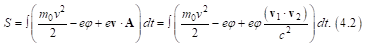In fact, the authors make an elementary error. If we consider the interaction of two charges, the scalar product of 4-speeds is:Indexes “1” and “2” refer to the first and second charge.The result obtained is invariant with respect to the Galilean transformation. Therefore, there is no need to introduce a fictitious momentum P into the Hamiltonian .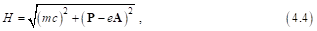whereExpression (4.4) actually does not contain the vector potential A.This “mathematical trick” requires special consideration.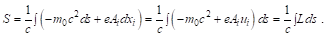Varying gives the following result.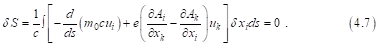The authors of  claim that by the arbitrariness of δx_i, the expression under the integral (4.7) is zero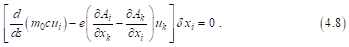From (4.8) the equation of motion follows (Lorentz formula for charge in the field):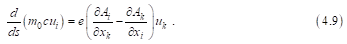It seems that everything is right and good. However, here it is not possible to get rid of the “ghosts”. It is enough to multiply any term in (4.1) by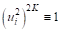or add to the Lagrangian a “zero term”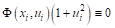and we will get “ghosts”,,, The authors understand that there are problems with the implementation of the principle of least action. They write that any term orthogonal to δxi can be added to the expression for the Lorentz force. You can, as in the first embodiment, consider examples. But this will not add new information.

The textbooks also set out other options for implementing the relativistic principle of least action (for example, ). We will not consider them because they have the same problems.

#### Orthogonality, but not Arbitrariness

To understand the causes of failures in the construction of the relativistic in-tegral of action, consider the general form of the variation of the relativistic inte-gral of action using the example of the second variant . Let us turn to the variation of the integral of the action (4.4)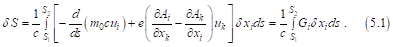The integrand is the scalar product of the “equation of motion” (the sum in square brackets or Gi) and the variation of the 4-vector δxi.  In  it is stated that “by virtue of the arbitrariness of the 4-vector δxi the equation of motion Gi) must be equal to zero”. The minimum of the integral of the action is realized on extremals determined from the solution of the "equation of motion" Gi = 0. In fact, this statement is either a mistake or a verbal adjustment to the desired result. Below we show it.

The equality of the scalar product to zero (Gi δXi = 0) does not at all imply that one of the factors is zero. The identity (Gi δXi = 0) does not change if we add to the “equation of motion” any term that is orthogonal to δXi, for example, ai. Then we have (Gi + ai) .δXi = 0. The authors are well aware of this fact and have written about it. The term ai. is the “ghost”, variants of which can also be included in the equation Gi = 0 itself.

One can offer another option. We write the variation of the integral action for this option.As in the previous case, we integrate the first term in the action integral by parts. Note thatThe first member of the right-hand side is equal to zero, since the ends of the trajectory S1 and S2 are rigidly fixed and the variation at these points is zero by the conditions of variation. The second term (integral) is identically zero since the linear operators d  and δ are commutative. The relativistic integral of action has no extremes. He is constant! The value of the integral depends only on the limits of integration and does not depend on the shape of the curve. The principle of least action does not hold.

Now we need to explain the reason for the constancy of the action integral. In classical mechanics, the action integral is chosen so that the Lagrange function is invariant with respect to the Galilean transformation.

Relativistic action integral is constructed by analogy. The relativistic Lagrange function must be invariant with respect to the Lorentz transformation. Therefore, it must be composed of true scalars. These true scalarscan beetc.

Consider a variation of the true scalar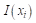, which for simplicity depends only on the 4-coordinate. Let the 4-coordinate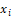get some incrementand becomes the 4-coordinate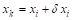On the one hand, the change in the true scalar is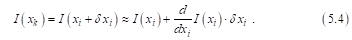On the other hand, we cannot arbitrarily change the 4-vector xi. Its change should be within the framework of the Lorentz transformation:, whereis the Lorentz transformation matrix. Under this condition, the true scalar cannot change. Consequently,So, any change in the true scalar is always orthogonal to the variation of the 4-vector δxi. The lengths of any 4-vectors in the Minkowski space are true scalars (invariants of the Lorentz transformation), i.e. quantities not dependent on the choice of the inertial reference system. By virtue of this, any 4-increment of a vector will always be orthogonal to a 4-vector.

Repeat. Since the relativistic Lagrange function is formed from invariants (true scalars), the variation of these invariants is always identically zero. This is the reason for the constancy of the relativistic integral of action, i.e. lack of extremes.

So, the variation of the relativistic integral of the action is always zero, i.e. The relativistic integral of the action has no extremes and its value depends only on the limits of integration!

An interesting is the physical interpretation of the constancy of the relativistic integral of action. The limits of integration S1 and S2 relativists suggest that we consider them as some “events” in 4-space. In fact, the limits S1 and S2  of the relativistic integral of action in 4-space are two concentric 4-spheres. The ends of the rectilinear segment S1 – S2 “abut” against these spheres. When varying, the ends of the segment freely slide along the indicated surfaces in space, touching them with their ends.

In the classical integral of action (as opposed to relativistic), the ends of the trajectory are rigidly “fixed” at fixed points t1 and t2, and only the shape of the trajectory varies, as is well known.

Comment. The above conclusions are partially valid for the action integrals that use the density of the Lagrange function to obtain field equations: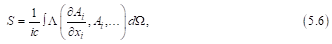where Λ is the density of the Lagrange function, dΩ is an elementary 4-volume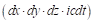. However, the situation changes if we present the integral of the action (5.6) in the following (classical) form: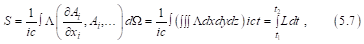whereL is the classical Lagrange function

As we wrote above, the variation of any invariant entering the relativistic Lagrange function is always orthogonal to the 4-vector δi. This circumstance is the cause of the appearance of “ghosts” in the equations of motion and ambiguity of equations.

Once again, we mention that talking about the relativistic principle of least action is pointless. The relativistic integral of action is constant and has no extremes. As a consequence, the equations for electromagnetic and gravitational fields, which were obtained using the relativistic principle of least action, are not only ambiguous, but also highly doubtful. The “elegant mathematical formalism”, which apologists of relativistic theories have always been so proud of, turns out to be incorrect in practice. It resembles “an elegant soap bubble.”

## Conclusion

So, we found that the mathematical formalism that implements the relativ-istic principle of least action is not correct. Consequently, the equations of mo-tion obtained within the framework of this principle are not unambiguous and correct.

The drama of the situation lies in the fact that many theoretical conclusions are being questioned, as well as interpretations of previously obtained experi-mental results. This concerns, first of all, electrodynamics, the theory of acceler-ators, the theory of elementary particles, etc. It is necessary to formulate and im-plement a new version of the principle of least action.

We analyzed the fundamentals of relativistic mechanics  and established the following facts:

1. In analyzing the Lorentz transformation, Einstein derived the correct for-mulas relating spatial intervals and time intervals in two inertial reference sys-tems (“scale compression” and “time dilation”).
2. However, he gave the wrong explanation for these phenomena. “Com-pression of scale” is connected not with “deformation of space”, but with dif-ferent directions of the front of the light wave in two inertial reference systems . "Slowing down time" is the transverse Doppler effect . The erroneousness of the Einstein explanation is seen by many authors. For example, O. D. Jefimenko privately told one of the authors (Andrew Chubykalo): ... if there is a reduction in the length of the moving body ... However, I am convinced that such a reduction does not exist, as explained in my book O. D. Jefimenko, “Eletromagnetic Retardation and Theory of Relativity”   and in Zeitschrift fuer Naturforschung: Oleg D. Jefimenko, “On the experimental proof of the relativistic length contraction and time dilatation ”, Z. Naturforsch. 53a, 977-982 (1998) .
3. Thus, we return again to the classical space-time representations. There are no contradictions between the Lorentz transformation and the Galilean transformation. The coefficients in the Lorentz transformation matrix (the relative velocity of the observer and the object) are invariant with respect to the Galilean transformation, since the relative velocity is an invariant of the Galilean transformation.

It is possible that the GTR expects a similar fate (See our work “The postlate ofthe equivalence of masses or the law of their proportionality?” .

Comment. We did not mention in vain in the Introduction about early logical positivism. Positivist philosophers reduced the role of philosophy to the methodology of science. By helping to hide the internal contradictions in physics, they actually created an atmosphere of intolerance for criticism of the SRT, GTR and other new theories. This circumstance created an atmosphere of dogmatism in physics. The “development” of philosophy in most cases was reduced to the multiplication of philosophical terms and scholasticism, but not to the analysis of the ideological aspects of physical theories. It is necessary to restore the materialist philosophy in physics.

 For example, in Russia, even now, the “Inquisition in Science” takes place. This is the Committee to combat pseudoscience. This Committee prevents the publication of articles criticizing the SRT, GTR and the discussion of alternative points of view.

## References

1. Chubykalo A, Kuligin V (2018) Unknown classical electrodynamics. BJMP 4: 384-425. [crossref]
2. Mario Augusto Bunge  (1973) Philosophy of Physics. Springer. [crossref]
3. Landau LD, Lifshitz EM (2010) The Classical Theory of Fields.[crossref]
4. Feynman R, Leighton R, Sands M (1977) The Feynman Lectures on Physics: Mainly Electromagnetism and Matter. 2 (Addison-Wesley). [crossref]
5. Goldstein H (1950) Classical Mechanics. (Cambridge, Addison Wesley). [crossref]
6. Panofsky W, Phillips M (1990) Classical Electricity and Magnetism. [crossref]
7. Chubykalo A, Espinoza A,  Kuligin V, et al. (2019) Why does the struggle around srt continue to this day?.  Int J Res- Granthalayah 7: 205-237. [crossref]
8.  Jefimenko O (2004)  Electromagnetic Retardation and Theory of Relativity. [crossref]
9. Jefimenko O (1998) Zeitschrift fuer Naturforschung 53: 977-982. [crossref]
10. Chubykalo A,  Espinoza A and  Kuligin V (2019) International journal of engineering sciences & research technology 8: 129-138.

Research Article

#### Publication history

Accepted date: October 29, 2019
Published date: November 02, 2019

© 2019 Chubykalo A. This is an open-access article distributed under the terms of the Creative Commons Attribution License, which permits unrestricted use, distribution, and reproduction in any medium, provided the original author and source are credited.

#### Citation

Chubykalo A, Espinoza A, Korneva M. (2019) Integral of action with the “ghosts”. OSP J Nuc Sci 1. JNS-1-104